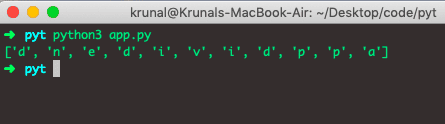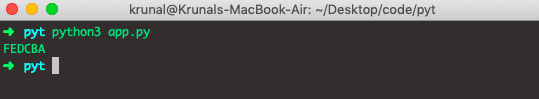# Python reversed: The Complete Guide

The reversed() method returns the iterator that accesses the given sequence in the reverse order.

## Python reversed()

Python reversed() is an inbuilt function that returns an iterator that accesses the given sequence in the reverse order. The iter() function returns an iterator object. The list.reverse() method reverses a List.

### Syntax

`reversed(seq)`

### Parameters

The sequence parameter is required, and it is an iterable object.

It could be an object that supports sequence protocol (__len__() and __getitem__() methods) as a tuplestringlist, or range.

See the following example.

```# app.py

# for string
string = 'AppDividend'
print(list(reversed(string)))

# for tuple
tuple = ('k', 'r', 'u', 'n', 'a', 'l')
print(list(reversed(tuple)))

# for range
range = range(19, 24)
print(list(reversed(range)))

# for list
listA = [19, 21, 46, 29, 6]
print(list(reversed(listA)))```

See the following example.In the above code example, we have used the string, range, list, and tuple data types and structures.

## How reversed works for custom objects

Let’s see an example of reversed with custom objects.

```# app.py

class AppDividend:
title = ['a', 'p', 'p', 'd', 'i', 'v', 'i', 'd', 'e', 'n', 'd']

def __reversed__(self):
return reversed(self.title)

obj = AppDividend()
print(list(reversed(obj)))```

See the output.We can supply the custom object as a reversed() function argument if it has the __reversed__() method or supports a sequence protocol.

We need to implement a __len__() method and the __getItem__() method with integer arguments starting at 0 to support the sequence protocol.

So, Python reversed() function returns the reverse iterator. The seq must be the object which has the __reversed__() method or supports a sequence protocol (the __len__() method and a __getItem__() method with the integer arguments starting at 0).

By using the reversed() function and a string join() function, you can reverse the string. For example, if we have the string “ABCDEF” and then if you apply the join() function, then you will get the reverse string. For more detail, see the how to reverse string example.

See the below example.

```# app.py

string="ABCDEF"
print(''.join(reversed(string)))```

See the output.That’s it for this tutorial.

This site uses Akismet to reduce spam. Learn how your comment data is processed.